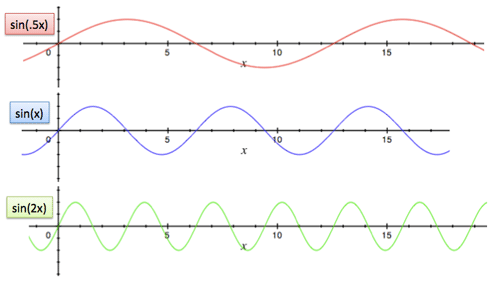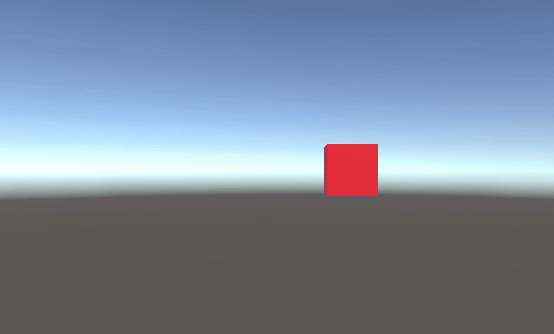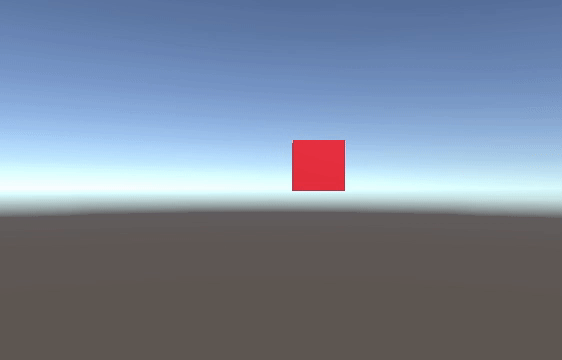Math is widely used in game development, and one of my favourite maths is sine wave. Don’t be scared of trigonometric functions because in this article I will only cover one super simple function namely sine. By using sine function, you will be able to create motions as below:

• Circular MotionImage comes from AT Game Studio

• Ping Pong Motion:Image comes from l.j-factor.com

You might wonder why can’t we just use a normal animation clip to animate a gameobject? The answer is that by applying sine function, we will be benefit from the flexibility of changing the animation speed and range. And I shall explain later.

### Preknowledge

First of all, let’s have a look of the sine wave as the figure below. With time(or x value) increasing, sine wave oscillates between -1 and 1 repetitively. This nice feature will help us to create a variety of animations.Secondly, the following image shows that by multiplying a constant to x value, we will be able to change the speed of the sine wave cycling.Image comes from Intuitive Understanding of Sine Waves

### Example

The next part of this article is to create an example in Unity and introduce how do we use sine function to achieve animations. Our objective is to create a cube in an empty scene, and let the cube do ping pong and circular movement with the ability to speed up/down the motion and increase/decrease the range of movement.

Formula: As sine function will result in a value between (-1, 1), by multiplying an amplitude value will update the range of the final result.

``````   f(x) = amplitude * Sin(speed * x)
``````

Ping-pong motion: let’s first create a ping-pong movement of the cube in our Unity project. Create a script called MathWave.cs and attach the script on the cube. The following script does 1 thing: which is updating the cube’s x position with sine function.

``````   public class MathWave : MonoBehaviour
{
float elapsedTime = 0f;
public float speed = 1f; // Default speed
public float amplitude = 1f; // Default range

void Update()
{
// Use Sine wave on cube's x position
float x = amplitude * Mathf.Sin(speed * elapsedTime);

// Update x position
transform.position = new Vector3(x,
transform.position.y,
transform.position.z);

// Increase time
elapsedTime += Time.deltaTime;
}
}
``````

If we run the project, you will see our cube is moving from left to right, right to left repeatedly. The reason is that sine wave repeatedly travels from 1 to -1 and -1 to 1.Circular motion: The coordinates x and y of any points in a unit circle have the relationship as the figure below.We can represent the coordinates (x, y) as ( cos(v), sin(v) ). Therefore, we can achieve circular motion by updating the x position using Cosine wave and y position using Sine wave.

``````void Update()
{
float x = amplitude * Mathf.Cos(speed * elapsedTime);
float y = amplitude * Mathf.Sin(speed * elapsedTime);
transform.position = new Vector3(x, y, transform.position.z);
elapsedTime += Time.deltaTime;
}
``````Lastly, we can change the speed value to speed up/down the motion, and change the amplitude value to increase/decrease the motion path.